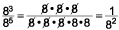## 1.2 Learn Basic Properties and Operations

This lesson will help you learn the basic properties and rules that govern math problem-solving.

### Exponents

The powers of ten form patterns that can help you understand exponents. In the examples, pay close attention to the pattern formed by the zeros.

### Examples

 $$\begin{array}{c}\,\,\,\,\,\,\,\,\,\,\,\,{10^4} = 10,000\\\,\,\,\,\,\,\,\,\,{10^3} = 1,000\\\,\,\,\,\,{10^2} = 100\\\,\,\,{10^1} = 10\\{10^0} = 1\end{array}$$

Did you notice? The powers of ten are very easy to figure out. Each is equal to 1 followed by the number of zeros shown by the exponent.

This pattern also reveals what will happen when we raise a number to the first or zero power.

Any number raised to a power of 1 doesn’t change: $${a^1} = a$$
Any number raised to a power of 0 equals 1: $${a^0} = 1$$

.

Exponents can also be negative numbers. You already know that to divide exponents, you should subtract.

### Examples

 $${\textstyle{{{8^3}} \over {{8^5}}}} = {8^{3 - 5}} = {8^{ - 2}}$$ You can also think of it this way:You can see that $${8^{ - 2}} = {\textstyle{1 \over {{8^2}}}}$$ . When you see a negative exponent, move the base and its exponent to the other side of the fraction bar and change the sign of the exponent.

### Examples

 $${3^{ - 2}} = {\textstyle{1 \over {{3^2}}}}$$ $${\textstyle{1 \over {{4^5}}}} = {4^{ - 5}}$$ $${\textstyle{{{2^{ - 2}}} \over {{9^{ - 3}}}}} = {\textstyle{{{9^3}} \over {{2^2}}}}$$
.